## Techniques for proving inequalities

### The articles

Compressions Quick description ( Suppose that a parameter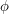(such as volume, for instance) is associated with a certain kind of mathematical object, and one would like to find the object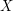for which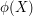is maximized. A useful general idea is to find a compression: that is, an operationthat takes an objectand produces a new object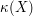with the property that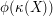is at least as big as, with equality if and only if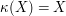. Then we know that anyfor whichis maximized must satisfy, which may well give us enough information aboutto allow us to solve the problem. )

Proving inequalities using convexity Quick description ( Jensen's inequality states that if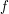is a convex function and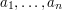are positive real numbers that add up to 1, then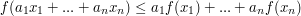. Many well-known inequalities follow from this one. More generally, convexity can be a powerful tool for proving inequalities. )

Quick description ( If you have two ways of calculating the same quantity, then you may well end up with two different expressions. If these two expressions were not obviously equal in advance, then your calculations provide a proof that they are. Moreover, this proof is often elegant and conceptual. Double counting can also be used to prove inequalities, via a simple result about bipartite graphs. )

Sums of squares Quick description ( If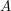and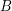are real numbers and you want to prove thatis at most as big as, then often a good way to do it is to express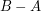as a sum of squares of real numbers. )

The tensor power trick Quick description ( If you want to prove that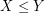, whereand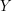are some non-negative quantities, but you can only see how to prove a quasi-inequality that says that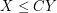for some constant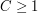, then try to replace all objects involved in the problem by "tensor powers" of themselves and apply the quasi-inequality to those powers. If all goes well, one can show that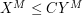for all positive integers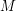, with a constant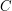which is independent of, which implies thatsince one can taketh roots and then lettend to infinity. )

Bounding probabilities by expectations Quick description ( Ifis a random variable and you would like a good upper bound for the probability that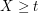, then a good way of doing so is sometimes to choose a non-negative increasing function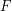and use the fact that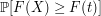is at most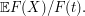This article is a discussion of the method. )